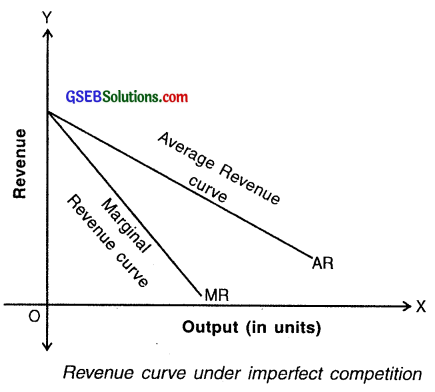# GSEB Solutions Class 11 Economics Chapter 5 Cost of Production and Concept of Revenue

GSEB Gujarat Board Textbook Solutions Class 11 Economics Chapter 5 Cost of Production and Concept of Revenue Textbook Exercise Important Questions and Answers, Notes Pdf.

## Gujarat Board Textbook Solutions Class 11 Economics Chapter 5 Cost of Production and Concept of Revenue

### GSEB Class 11 Economics Cost of Production and Concept of Revenue Text Book Questions and Answers

1. Choose correct option for the following from the options provided :

Question 1.
How is Average Cost curve shaped?
(A) Hockey-stick
(B) U
(C) V
(D) Square
(B) U

Question 2.
Which cost cannot be measured?
(A) Real cost
(B) Monetary cost
(C) Opportunity cost
(D) Long run cost
(A) Real cost

Question 3.
When production is zero then which cost is positive?
(A) Monetary cost
(B) Average cost
(C) Variable cost
(D) Fixed cost
(C) Variable cost

Question 4.
Which cost has direct relation with the production units?
(A) Fixed cost
(B) Variable cost
(C) Average cost
(D) Marginal cost
(B) Variable cost

Question 5.
In which market, Average Revenue and Marginal Revenue are same?
(A) Perfect Competition
(B) Monopoly
(C) Monopolistic Competition
(D) Oligopoly
(A) Perfect CompetitionQuestion 6.
How is the slope of fixed cost curve?
(A) Negative
(B) Positive
(C) Parallel to X-axis
(D) Parallel to X-axis
(C) Parallel to X-axis

2. Answer the following questions in one sentence :

Question 1.
Why does the average fixed costs decrease with the increase in production?
When production increases the Total Fixed Cost (TFC) gets distributed among more units and so Average Fixed Cost decreases with increase in production.

Question 2.
Give formula of Marginal cost.
Marginal Cost (MCn) = Tcn – Tc(n-1), Here, n = no. of units produced and Tc = Total cost.

Question 3.
What do you mean by fixed cost? How is the fixed cost curve?
In a short period (run), the production may increase, decrease or become zero i.e. no production, but the cost would remain same. Such a type of cost is called the fixed cost. Fixed cost is also known as overhead cost. The fixed cost curve is parallel to X-axis.

Question 4.
Which concept of revenue can be known as price?
The Average Revenue curve shows the Average Revenue of a producer by selling of commodity.

Question 5.
What do you mean by Marginal Revenue?
The change in total revenue which results from the sale of one more unit of a commodity is called the marginal revenue. Formula : MRn = Rn – R(n – 1)

Question 6.
What do you mean by short run?
A short term (run) is a time period in which a producer cannot change factors of production. Hence in this period all the factors of production such as plant, heavy machinery, building of a factory, etc. remain fixed.

Question 7.
What is opportunity cost?
The means of production have alternative uses i.e. more than one use. The concept of opportunity cost is based on the particular characteristic of factor of production which says that when a factor is used for a particular use, the other use is left out or the same cannot be used for other purpose. Under such circumstances, the best alternative which remains is called the opportunity cost of production.

Question 8.
What is monetary cost?
Generally, the amount that the producer pays monetarily for the process of production is called its monetary cost. Thus, the cost of production in terms of money is known as monetary cost. Monetary cost includes wages, rent, raw material, fuel and the total of all the expenditure made by the producer.]

Question 9.
What does the firm get when marginal cost is less than Marginal Revenue?

Question 10.
What is real cost?
According to Marshall, the labourers, capitalists and entrepreneurs who are involved in the process of production bear psychological and physical burden. Such burden is called real cost.

3. Answer the following questions in short :

Question 1.
What do you mean by short run?
Short term (run):

• A short term (run) is a time period in which a producer cannot change factors of production. Hence in this period all the factors of production such as plant, heavy machinery, building of a factory, etc. remain fixed.
• The producer can increase/decrease production by increasing or decreasing variable factors such as raw material, labour, electricity, etc.
• Moreover, the producer cannot change the size of firm but can increase production by increasing the capacity of factors of production.

Long term (run):

• A long term (run) is a time period in which a producer can change all the factors of production. Hence, in this period all the factors of production such as plant, heavy machinery, building of a factory, etc. are considered variable.
• The producer can increase or decrease these factors of production and hence increase/decrease the production in long term (run).
• The producer can change the size of the firm. By doing so he can change the total production to a large extent in a long term. The firm can also expand its size by using new and modern technology.

Question 2.
What is the meaning of average fixed cost? Give example.
Average Fixed Cost (AFC):
The cost of per unit of output is called the Average Fixed Cost (AFC). AFC is obtained by dividing total fixed cost of a firm with total production unit.

∴ Average Fixed Cost (AFC) = $$\frac{\text { Total Fixed Cost (TFC) }}{\text { Total Production Unit (TP) }}$$

Thus, AFC = $$\frac{\mathrm{TFC}}{\mathrm{TP}}$$

Example:

• Fixed cost of a company is ₹ 50,000. If the producer produces 1000 units of a good then,
Average Fixed Cost = $$\frac{50000}{1000}$$ = ₹ 50
• Thus, on an average the company bears ₹ 50 as fixed cost on each unit produced.
 Output (units) Total Fixed Cost (in ₹) [TFC] Average Fixed Cost (in ₹) [TFC/output] 10 100 10 20 100 5 30 100 3.3 40 100 2.5 50 100 2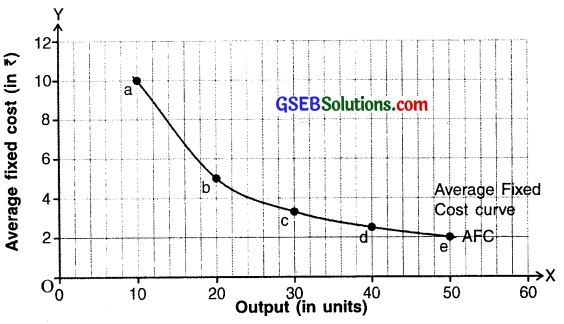Diagram of average fixed cost

Graph and curve:

• Units produced are shown on X-axis whereas average fixed cost (AFC) is shown in ₹ on Y-axis.
• When production increases the Total Fixed Cost (TFC) gets distributed among more units and so Average Fixed Cost decreases. Due to the decreasing cost, the average fixed cost curve (AFC) is a downward sloping curve, but it never becomes zero.Question 3.
‘All costs are variable in the long run.’ Explain.

• Variable cost increases if cost of production increases and decreases if the cost of production decreases and also becomes zero if production is zero.
• This cost has direct (positive) relation with quantity of production.
• Price of raw material, energy consumption, transportation expenditure, labour wages, tax on product, sales tax, etc. are variable costs which are directly dependant on the quantity of production.
• All these costs affect the production only in short run. In the long run , changes can occur in all of these.
• For example, there might occur a huge change in price of raw material, mode of transportation may change, taxes may be added or abolished. Similarly, fixed costs might also vary largely. The producer may buy new premises, change his machinery, increase labour, etc.
• Hence, irrespective if the cost is fixed or variable, it becomes variable in the long run.

Question 4.
Give meaning of total cost and total revenue.
1. Total Cost (TC):

• The summation of Total Fixed Cost (TFC) and Total Variable Cost (TVC) is called Total Cost (TC).
• Therefore, TC = TFC + TVC

2. Total Revenue (TR):

• Money received by a firm on selling the units it produces is known as revenue. The total income received by firm from sale is called total revenue. This income is known as total revenue or sale revenue.
• A firm’s total income is based on two things:
1. Price per unit and
2. Total sale.
• Change in one or both the factors brings change in the revenue of the firm.

Formula:
Total Revenue (TR) = Units sold (Q) × Price of commodity (P)

Question 5.
Why is the revenue curve negatively sloped in imperfect competition?

• In imperfect market competition, the seller has to reduce the price in order to sell more units.
• This means he tries to increase demand of his commodity by increasing its sale (by decreasing prices). So, his total revenue increases at a diminishing rate.
• This results in a difference between the Average Revenue (AR) and Marginal Revenue (MR).
• As price decreases, the Average Revenue decreases and its curve slopes downwards from left to right. Hence, the revenue curve is negatively sloped.

4. Answer following questions to the point :

Question 1.
Give the meaning of fixed cost and explain with the help of diagram.
Fixed cost (FC):

• In a short period (run), the production may increase, decrease or become zero i.e. no production, but the cost would remain same. Such a type of cost is called the fixed cost. Fixed cost is also known as overhead cost.
• Thus, in short period there is no relation between fixed cost and quantity of production.
• Fixed cost includes salary of permanent staff, rent of factory building, house- and property tax, license fee, interest on capital, premium of insurance, etc.

Example:
The schedule shows production of pens for a firm and its fixed cost.

 Units of output Total Fixed Cost ( ₹) 0 100 10 100 20 100 30 100 40 100 50 100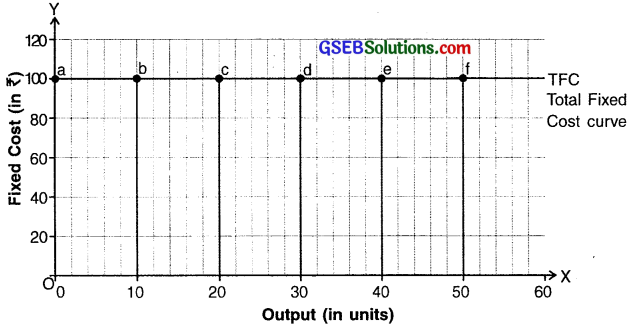Diagram of total fixed cost
As can be seen in schedule, irrespective of the rise in production of pen i.e. 10, 20, 30, 40 or 50, the production cost remains the same or say fixed at ‘100. This cost is called fixed cost or total fixed cost (TFC). Note that even with the production is 0, the producer has to bear the fixed cost.

Graph and curve:
As shown in the diagram, the output is measured on X-axis and total fixed cost in ₹ on Y-axis. Since, the Total Fixed Cost (TFC) remains constant, the TFC curve is parallel to X-axis.

Question 2.
Give the meaning of variable cost and explain with the help of diagram.
Variable cost (VC):

• In short term if the cost of production changes with the change in quantity of production it is called variable cost. The variable cost increases if cost of production increases and decreases if the cost of production decreases and also becomes zero if production is zero.
• Variable cost is also known as unstable or direct or main cost. This cost has direct (positive) relation with quantity of production and hence Prof. Marshall calls variable cost as a main cost.
• Price of raw material, energy consumption, transportation expenditure, labour wages, tax on product, sale tax, etc. are variable costs which are directly dependant on the quantity of production.
• The difference between fixed cost and variable cost is possible in short
period of time only. In a long run (period) all costs are variable costs.

Example:

• As shown in the schedule, when production is 0, the total variable cost (TVC) is also 0.
• As production increases, variable costs also increase. Although up to 30 units variable cost increases at a diminishing rate. The reason for this is that it is affected by the law of ‘increasing returns to scale’
• After 30 units, the law of ‘decreasing return to scale’ is applicable and so variable cost increases at increasing rate.
 Units of output Total Variable Cost (₹) 0 0 10 80 20 150 30 210 40 290 50 390

Graph and curve:

• As shown in diagram, output (in units) is measured on X-axis and total variable cost i.e. TVC (in ₹) is measured on Y-axis.
• As production increases from 10, 20, 30, the total variable cost also increases from 80, 150, 210 and so on. Hence, the total variable cost has positive slope from origin.
• In the beginning, variable cost increases at decreasing rate. Once it reaches the optimum level, the variable cost increases at increasing rate.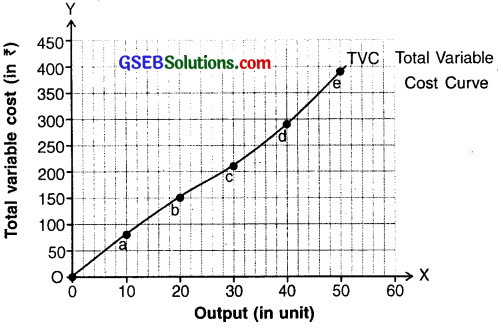Diagram of total variable costQuestion 3.
State the limitations in measuring opportunity cost.
Opportunity cost:

• The concept of opportunity cost was presented by Austrian economist but it was properly presented by Marshall. We know that the means of production have alternative uses i.e. more than one use. The concept of opportunity cost is based on the particular characteristic of factor of production which says that when a factor is used for a particular use, the other use is left out or the same cannot be used for other purpose. Under such circumstances, the best alternative which remains is the opportunity cost of production.
• If a factor of production is used in the production of one commodity which seems the best, the next best or say the second best alternative is left out.
• Assuming the best choice is made, opportunity cost is the ‘cost’ incurred by not enjoying the benefit that would have been had by taking the second best available choice

Example:
(a) If someone is producing wheat on one piece of land, then at the same time on the same piece of land other food grain (crop) cannot be produced.

(b) A worker working in textile mill cannot at the same time work in any other industry.

• Suppose if wheat is produced on a piece of land one can earn an income of ₹ 2 lakh can be earned and if rice is produced the income of ₹ 3.5 lakh can be earned.
• The farmer decides produce rice in which he earns more.
• So, to get the income of ₹ 3.5 lakh from the production of rice, farmer loses out income of ₹ 2 lakh from the production of wheat. This left out income of ₹ 2 lakh from the production of wheat is called the opportunity cost of ₹ 3.5 lakh earned from the production of rice.

Problems in measuring opportunity cost:
(I) Factors with one use:
If a factor of production has only one use then its opportunity cost cannot be decided.

Example:
(a) Suppose if a piece of land is used only to produce grass so far than we cannot calculate the opportunity cost of that land.
(b) The same applies for a person who is currently unemployed. Since the person does not have any work how can we calculate alternative cost?

(II) Factors having specific use:
If factors of production have only a specific use then the concept of opportunity cost is not useful. Returns of these factors are not decided by their alternative uses but on the basis of their demand.

Example:
(a) Persons having expertise over computers, scientist having knowledge of atomic power, etc. These people do not know any other work except their own.
(b) Machine for making ice can only produce ice i.e. it has no alternative use and so no opportunity cost is involved.

5. Answer the following questions in detail :

Question 1.
Explain different concepts of the cost of production.
There are three important concepts regarding cost of production. They are:

1. Real cost
2. Opportunity cost and
3. Monetary cost.

1. Real cost:

• According to Marshall, the labourers, capitalists and entrepreneurs who are involved in the process of production bear psychological and physical burden. Such burden is called real cost.
• Money spent by producers for production work of goods is not the only cost of production. Mental factors such as fatigue, boredom tension, stress faced by the labourers, the anxiety faced by entrepreneur or investors who risk their saving and capital, insecurity of wrong decisions, etc. are also the factors included in the real cost.
• Owing to the monetary as well as various other factors, real cost cannot be actually measured in monetary terms. Hence, real cost is also called non-monetary cost.
• As per Marshall, the factors of production have to bear this real cost. So, to attract these factors return is given in the form of wage, interest and profit.

Problems in measuring real cost:

• As per the concept of real cost psychological factors such as fatigue, boredom, pain, sacrifice and anxiety are a part of production of goods. It is quite
difficult to measure the real cost of goods which face these psychological factors during production.
• Moreover, other factors such as the smoke emitted by factories, the polluted water released in rivers, streams, etc. create adverse effect on health of the people of surrounding area. From the perspective of society this adverse effect is also a cost which cannot be measured.

2. Opportunity cost:

• The concept of opportunity cost was presented by Austrian economist but it was properly presented by Marshall. We know that the means of production have alternative uses i.e. more than one use. The concept of opportunity cost is based on the particular characteristic of factor of production which says that when a factor is used for a particular use, the other use is left out or the same cannot be used for other purpose. Under such circumstances, the best alternative which remains is the opportunity cost of production.
• If a factor of production is used in the production of one commodity which seems the best, the next best or say the second best alternative is left out.
• Assuming the best choice is made, opportunity cost is the ‘cost’ incurred by not enjoying the benefit that would have been had by taking the second best available choice

Example:
(a) If someone is producing wheat on one piece of land, then at the same time on the same piece of land other food grain (crop) cannot be produced.

(b) A worker working in textile mill cannot at the same time work in any other industry.

• Suppose if wheat is produced on a piece of land one can earn an income of ₹ 2 lakh can be earned and if rice is produced the income of ₹ 3.5 lakh can be earned.
• The farmer decides produce rice in which he earns more.
• So, to get the income of ₹ 3.5 lakh from the production of rice, farmer loses out income of ₹ 2 lakh from the production of wheat. This left out income of ₹ 2 lakh from the production of wheat is called the opportunity cost of ₹ 3.5 lakh earned from the production of rice.

Problems in measuring opportunity cost:
(I) Factors with one use:
If a factor of production has only one use then its opportunity cost cannot be decided.

Example:
(a) Suppose if a piece of land is used only to produce grass so far than we cannot calculate the opportunity cost of that land.
(b) The same applies for a person who is currently unemployed. Since the person does not have any work how can we calculate alternative cost?

(II) Factors having specific use:
If factors of production have only a specific use then the concept of opportunity cost is not useful. Returns of these factors are not decided by their alternative uses but on the basis of their demand.

Example:
(a) Persons having expertise over computers, scientist having knowledge of atomic power, etc. These people do not know any other work except their own.
(b) Machine for making ice can only produce ice i.e. it has no alternative use and so no opportunity cost is involved.

3. Monetary cost:
Generally, the amount that the producer pays monetarily for the process of production is called its monetary cost. Thus, the cost of production in terms of money is known as monetary cost. Monetary cost includes wages, rent, raw material, fuel and the total of all the expenditure made by the producer.

Example:

• If a factory producing pens incur the cost of ₹ 50,000 to produce 1000 units of pen, the monetary cost to produce 1,000 units of pen is ₹ 50,000.
• Real cost and opportunity cost have many limitations which make their calculation very difficult. Hence, the concept of monetary cost is widely used in economic analysis, for decisions related to production and in price determination. Since cost of production is calculated in terms of money, the concept of monetary cost is important.Question 2.
Explain with diagram the inter-relationship between average cost and marginal cost.
Inter-relationship between Average Cost and Marginal Cost:

• The relationship between the average cost and the marginal cost is quite important for studying production cost.
• We know that cost per unit of output is called Average Cost (AC) and the cost increased to produce one extra unit of commodity is called the Marginal cost (MC).

A producer in long run decides to continue production if:
Price of a commodity is more than its Average Cost (AC).

On the other hand, in short run, the producer decides to continue production if:

• Price of commodity is more than Marginal Cost (MC).
• Thus, the Average Cost (AC) and the Marginal Cost (MC) play important role in taking a decision about production.

Example :
The table below shows Total cost, Average Cost and Marginal Cost of a firm for an output of a particular commodity.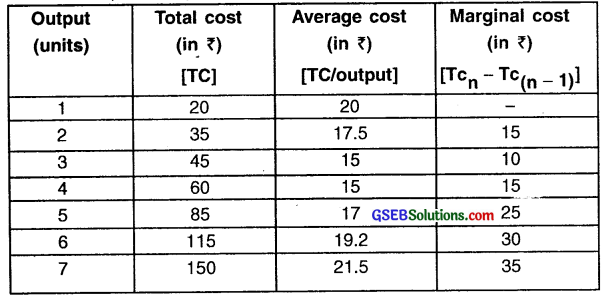• As shown in the schedule, as output increases, initially the Average Cost (AC) and the Marginal Cost (MC) both decrease upto 3rd unit produced because of the law of increasing returns to scale is applicable.
• At the 4th unit of output Average Cost and the Marginal Cost both become equal. Moreover, AC is minimum at 4th unit.
• After this point due to the decrease returns to scale law, both AC and MC increase.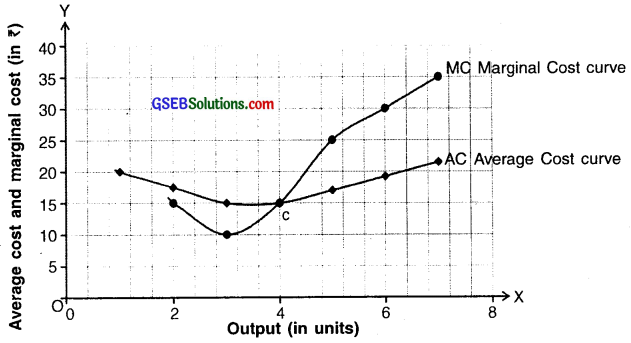Diagram of relationship between average cost and marginal cost

Diagram: The output unit is shown on X-axis. Average Cost (AC) and Marginal Cost (MC) are shown on Y-axis and their curves are plotted.

Relation between AC and MC:
1. Marginal Cost < Average Cost (MC < AC):
Initially, as average cost decreases marginal cost also decreases but, marginal cost decreases more rapidly than the Average Cost. Hence, when marginal cost is decreasing its curve remains below the curve of average cost i.e. MC < AC.

2. Marginal Cost = Average Cost (MC = AC):
When Average Cost is minimum, the Marginal Cost curve intersects the Average Cost curve from below. At the point of intersection the Marginal Cost and Average Cost become equal i.e. MC = AC.

3. Marginal Cost > Average Cost (MC > AC):

• When Marginal Cost curve intersects the Average Cost curve, both the costs start increasing. After this point increase in Marginal Cost is rapid than the increase in Average Cost.
• Hence, Marginal Cost curve goes above Average Cost curve i.e. MC > AC.

Question 3.
Explain Average Revenue and Marginal Revenue with the help of diagram in perfect competition market.
Perfect competitive market:
1. Perfect market or perfect competitive market is defined by several characteristics.

Some are:

• Perfectly competitive market is such a market where firms accepts market price and sell their commodities
• Commodities are homogeneous (i.e. the qualities and characteristics of goods or services available in market do not vary between different suppliers)
• There are large number of buyers and sellers
• Buyers and sellers have complete knowledge of market situation
• Price is determined by demand and supply of a commodity. Firms sells commodity on this price only and no firm can influence this price. Hence, price is fixed and constant.

2. In perfect competition, market price (P) = Average Revenue (AR) = Marginal Revenue (MR) i.e. (P – AR = MR).

3. Under such market condition, if price of commodity is ₹ 50 then Average
Revenue and Marginal Revenue of firm will also be ₹ 50.

4. As a result, the curves of Average Revenue and Marginal Revenue of the firm are same and also parallel to X-axis. This curve is represented as DD in the diagram.

In the diagram, revenue curve of a firm is shown. We can see three 7 things in it. They are:

1. In perfectly competitive market, Marginal Revenue and Average revenue are constant and same. As a result, both of them can be shown through one line or say curve DD.
All points on DD curve will show Average Revenue = Marginal Revenue.
2. Average Revenue and Marginal Revenue curve are parallel to X-axis as shown by DD curve. Here, Average Revenue and Marginal Revenue curve have merged into one another and since value of both the revenues are same and constant the slope of curve is zero.
3. Total Revenue curve represented by points ‘OR’ is at 45° angle at zero point i.e. origin point. This curve indicates that as the sale of goods increases, total revenue also increases at equal rate. Hence, the slope of total revenue curve is positive and of equal proportion.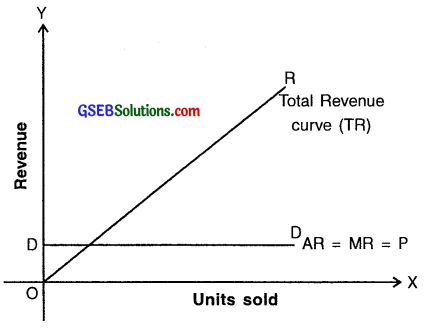Revenue curve in perfectly competitive market

Question 4.
Explain Average Revenue and Marginal Revenue with the help of diagram in imperfect competition market.